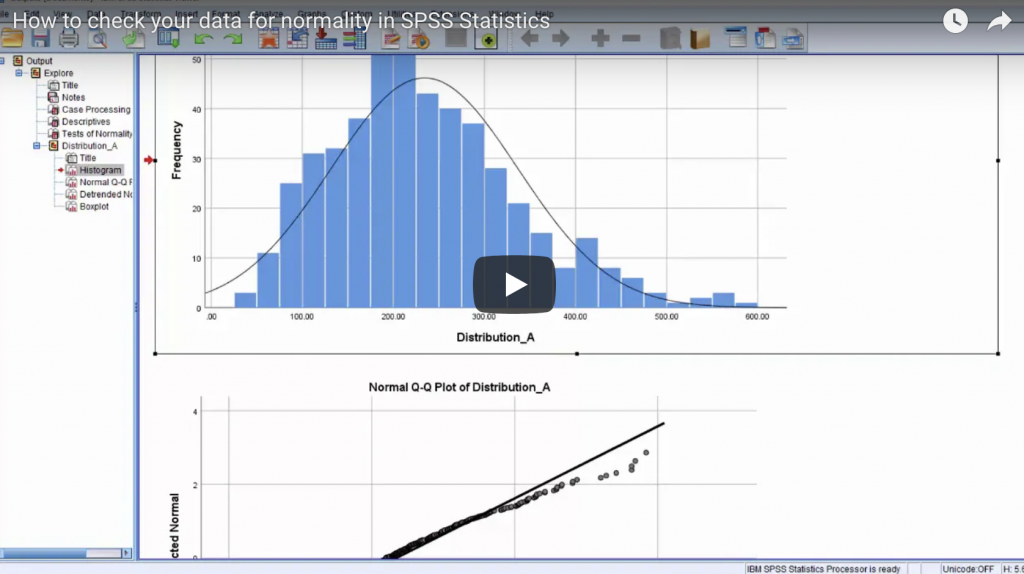# How to check your data for normality in SPSS StatisticsWhen you’re deciding which tests to run on your data it’s important to understand whether your data is normally distributed or not, as a lot of standard parametrical tests assume a normal distribution whereas other non-parametric tests are designed to be run on data which is not normally distributed. A normal distribution has a number of characteristics:-

• It is symmetrical
• It is bell-shaped
• Its mean, median and mode all appear at the same place
• Normal distributions can be divided up into the same proportions by the standard deviations, so 95% of the area under the curve lies within roughly plus or minus two standard deviations of the mean

In this video Jarlath Quinn demonstrates how to use the functions within the explore command in SPSS Statistics to test for normality.

Scroll to Top